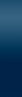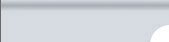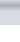MenuToolbarConstraints/ResultsImpedance SpectraEquivalent CircuitSettings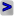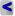EIS Spectrum Analyser Help Menu: Edit Multiply by Constant Use this option to normalise an EIS spectrum by electrode surface area. Normalise by Zmax Normalises an EIS spectrum by a maximal impedance magnitude. Subtract Element Subtracts the impedance of an element, which is connected in series to the main part of the circuit. This option allows subtracting e.g. resistance of an electrolyte solution from the spectrum. Select the element in the Subtract element dialog box, set the values of the corresponding parameters, and click OK button. Subtract EEC (series) Subtract EEC (parallel) Subtracts the impedance of a sub-circuit, which is connected in series or parallel to the rest of the circuit. Use Subtract EEC (series) e.g. to subtract a sub-circuit related to the grain boundaries from a spectrum of solid electrolyte. Assemble a sub-circuit to be subtracted, set the corresponding parameter values in the Result / In.Val. column, and select Edit / Subtract EEC from the main menu. EIS Spectrum Analyser, 2008-13